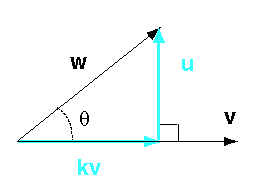Does a scaled vector have the same orientation as the vector?

Yes — scaling changes the length of a vector, but not its orientation.

# Projection of one Vector onto AnotherIn the diagram w and v are any two vectors. We want a vector u that is orthogonal to v. And we want scalar k so that:

w = kv + u

Then kv is called the projection of w onto v.

### QUESTION 4:

The vector u is orthogonal to v. What is true of u · v?